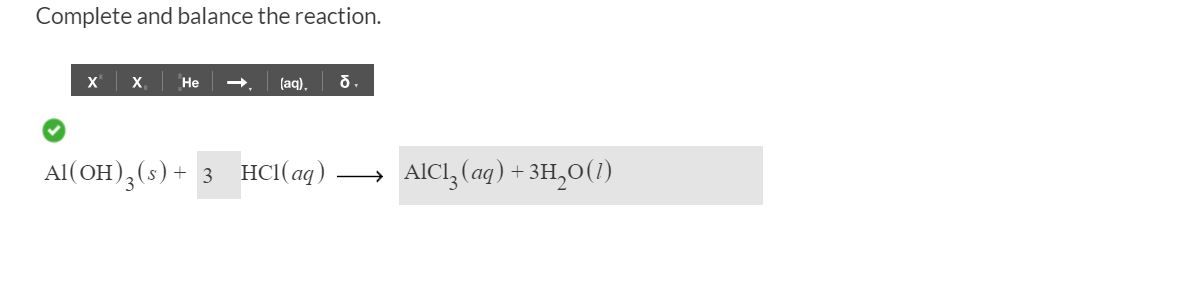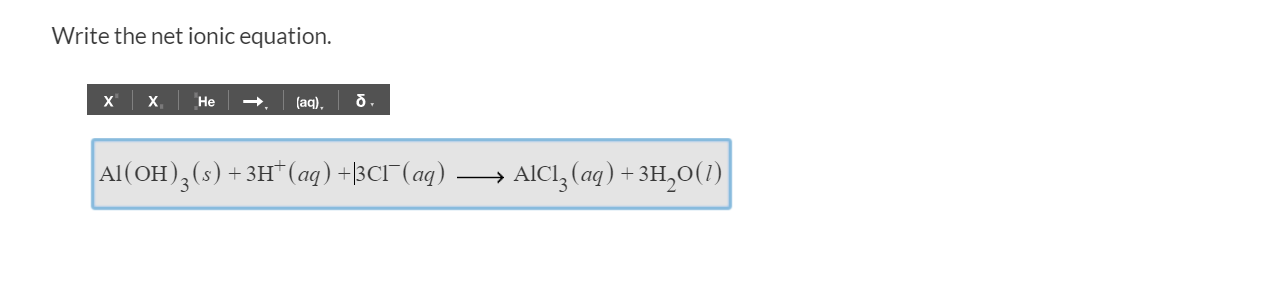# Complete and balance the reaction.XXНе(aq)Al(OH) (s)AICI, (aq) +3H20()3 HC(ад) Write the net ionic equationх.Не(aq)Al (OH)3 (s) +3H* (aq) +|3CI (aq) .. AICI, (ag) +3H20(1)|

Question
27 views

So I have figured out the right balanced equation in the first picture but I cannot figure out the net ion equation. I know that I do not include the spectator ions in a net ionic equation and to me the spectator ions would be the hydroxide ions (if Al(OH)3 dissociated in water) and the H+ ions but since aluminum hydroxide does not dissociate then it must be included in the net ion equation as Al(OH)3 so I would think that the net ion equation would be

Al(OH)3(s) + 3Cl-(aq) -> AlCl3(aq)

but that was the first thing I tried and it was wrong, so I tried adding in the 3H2O that gets made but that was wrong, so I added in the H+ ions and that still wasn't right, I cannot see where I am going wrong here. Please help!help_outlineImage TranscriptioncloseComplete and balance the reaction. X X Не (aq) Al(OH) (s) AICI, (aq) +3H20() 3 HC(ад) fullscreenhelp_outlineImage TranscriptioncloseWrite the net ionic equation х. Не (aq) Al (OH)3 (s) +3H* (aq) +|3CI (aq) . . AICI, (ag) +3H20(1)| fullscreen
check_circle

star
star
star
star
star
1 Rating
Step 1

The complete balanced equation between aluminium h...

### Want to see the full answer?

See Solution

#### Want to see this answer and more?

Solutions are written by subject experts who are available 24/7. Questions are typically answered within 1 hour.*

See Solution
*Response times may vary by subject and question.
Tagged in

### Inorganic Chemistry